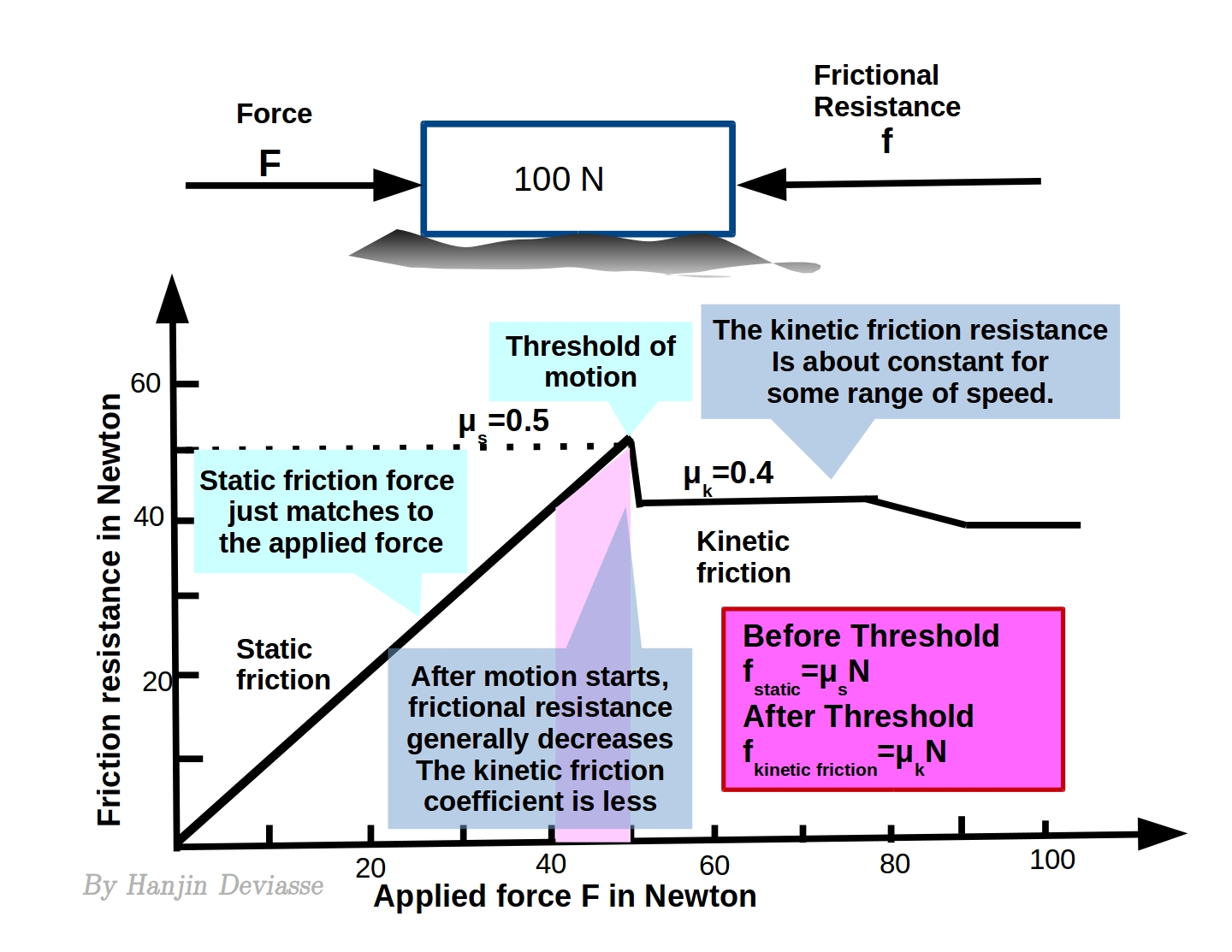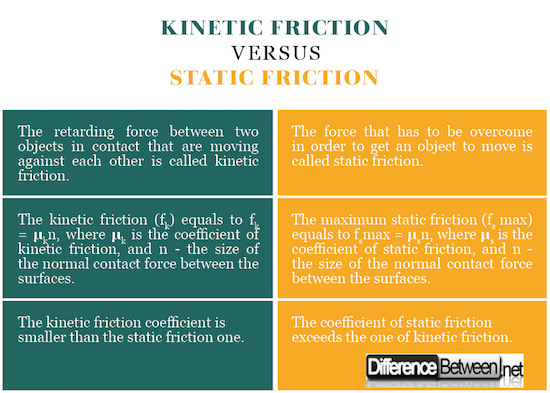#Difference Between Similar Terms and Objects

# Difference Between Kinetic Friction and Static Friction

The tangential component of the force of interaction between two surfaces in contact is called friction. It leads to resistance against movement between the surfaces and can cause mechanical deformation and heating.

Depending on whether the surfaces are at rest or in relative motion against each other, the friction divides into static and kinetic friction.## What is Kinetic Friction?

Kinetic friction is the retarding force between two objects in contact that are moving against each other. It depends on the type of the contacting surfaces. Kinetic friction is high for rough and dry surfaces and low for wet and smooth ones.

The force necessary to induce motion (i.e. to overcome the static friction), is bigger than the one necessary to continue the motion (i.e. to overcome kinetic friction). So the coefficient of kinetic friction (μk) is lower than the one of static friction (μs).

Kinetic friction remains constant between two surfaces, regardless of the relative speed of their movement. The coefficient of kinetic friction has a constant value for each pair of contacting surfaces (materials). For example it is 0.57 for steel / steel contact, 0.47 for steel / aluminum contact, etc.

The kinetic friction (fk) equals to:

fk = μkn,

where μk is the coefficient of kinetic friction,  and n – the size of the normal contact force between the surfaces.## What is Static Friction?

The force that has to be overcome in order to get something to move is called static friction. This is the force that prevents an object, placed on a sloped surface, from sliding.

At solid surfaces, the static friction occurs as a consequence of the surface roughness of the objects in contact. Its value depends on the type of the contacting surfaces. It is higher for rough and dry surfaces and lower for wet and smooth ones.

The force necessary to induce motion (i.e. to overcome the static friction), is bigger than the one necessary to continue the motion (i.e. to overcome kinetic friction). So the coefficient of static friction (μs), exceeds the one of kinetic friction (μk).

The coefficient of static friction has a constant value for each pair of contacting surfaces (materials). For example it is 0.74 for steel / steel contact, 0.61 for steel / aluminum contact, etc.

In order to make a stationary object move, we have to overcome the static friction force by an applied force. When a small force is applied to a nonmoving object, the static friction is of equal magnitude, but in the opposite direction to the applied force. When the force is being increased, at a certain point it reaches the maximum static friction value. At that point, the static friction is overcome and the object starts to move.

The maximum static friction (fs max) equals to:

fsmax = μsn,

where μs is the coefficient of static friction, and n – the size of the normal contact force between the surfaces.

## Difference Between Kinetic and Static Friction

### 1) Definition  of Kinetic and Static Friction

Kinetic  Friction: The retarding force between two objects in contact that are moving against each other is called kinetic friction.

Static Friction: The force that has to be overcome in order to get something to move is called static friction.

### 2) Formula for Kinetic and Static Friction

Kinetic friction: The kinetic friction (fk) equals to fk = μkn, where μk is the coefficient of kinetic friction,  and n – the size of the normal contact force between the surfaces.

Static friction: The maximum static friction (fs max) equals to fsmax = μsn, where μs is the coefficient of static friction, and n – the size of the normal contact force between the surfaces.

### 3) Magnitude of Kinetic and Static Friction

Kinetic Friction: The force necessary to induce motion is always bigger than the one necessary to continue the motion. So the kinetic friction coefficient is smaller than the static friction one.

Static Friction: The coefficient of static friction exceeds the one of kinetic friction.## Summary of Kinetic and Static Friction:

• The tangential component of the force of interaction between two surfaces in contact is called friction. It leads to resistance against movement between the surfaces and can cause mechanical deformation and heating.
• The retarding force between two objects that are moving against each other is called kinetic friction. The force that has to be overcome in order to get something to move is called static friction.
• Friction depends on the type of the contacting surfaces. It is high for rough and dry surfaces and low for wet and smooth ones.
• The force necessary to induce motion (i.e. to overcome the static friction), is bigger than the one necessary to continue the motion (i.e. to overcome kinetic friction). So the coefficient of static friction (μs), exceeds the one of kinetic friction (μk).
• The kinetic friction (fk) equals to fk = μkn, where μk is the coefficient of kinetic friction,  and n – the size of the normal contact force between the surfaces in contact. The maximum static friction (fs max) equals to fsmax = μsn, where μs is the coefficient of static friction, and n – the size of the normal contact force between the surfaces in contact.

Latest posts by Dr. Mariam Bozhilova Forest Research Institute, BAS (see all)

### Search DifferenceBetween.net :

Custom Search

Help us improve. Rate this post!Loading...Email This Post : If you like this article or our site. Please spread the word. Share it with your friends/family.

### 1 Comment

1. Thanks for the video

Please note: comment moderation is enabled and may delay your comment. There is no need to resubmit your comment.

## References :

Brown, R. Introductory Physics I: Elementary Mechanics. Morrisville: Lulu Press. 2013. Print.

Halliday, D. Resnick. Fundamentals of Physics Textbook. Hoboken: Wiley. 2004. Print.

Kuchling, H. Physics. Moscow: Mir. 1982. Print.

Image credit: https://commons.wikimedia.org/wiki/File:Static_Friction_%26_Kinetic_Friction.jpeg#/media/File:Static_Friction_%26_Kinetic_Friction.jpeg

Image credit: https://en.wikipedia.org/wiki/File:Static_kinetic_friction_vs_time.png#/media/File:Static_kinetic_friction_vs_time.png

Articles on DifferenceBetween.net are general information, and are not intended to substitute for professional advice. The information is "AS IS", "WITH ALL FAULTS". User assumes all risk of use, damage, or injury. You agree that we have no liability for any damages.